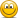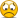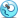# Exam FeedbackNovember 2018 Part 1 Exam Feedback

#### Nicole Seaman

##### Director of FRM Operations
Staff member
Subscriber
We hope that everyone did well on the FRM Part 1 exam on Saturday!We would love to hear any feedback that you have about the exam. How did it go? Did you encounter unexpected questions? Thank you in advance for any feedback you can provide!

#### DesNewman

##### Member
I thought the exam was tough but fair - I felt like the calculation questions were reasonable and the BT questions greatly prepared me for those as they felt like a breeze in comparison.

However, I felt like I really battled with the theorythere was far more of it than expected and I often couldn't see the differences between some of the choices offered, particularly all the theory questions around CCPs. I hope the calculation questions get me through - I have been so worried that the theory will be my down fall due to the tricky wording.

#### e2hope

##### New Member
In terms of Part 1, it was much easier than practice exam on GARP site.
I was rather perplexed to see there were not many calculation questions but lots of questions which asks delicate concepts.

#### Raisin

##### New Member
There is one question about sharpe ratio
it gives standard Deviation
Covariance=0.0009
Expected return =12%
Expected return =8%
Value 40000
Value 60000
Risk free rate=2.5%

#### Razi

##### Member
agreed with @e2hope the exam was much simpler than the practice exam offered by GARP but as @DesNewman said in theory question it often came down to two options that seem convincing so that maybe a potential pitfall for the candidates. Hoping for the best.

#### harshjain

##### New Member
On an average did everyone manage to complete the paper in full. For example I had to leave 14 questions

#### DesNewman

##### Member
I found there was enough time, simply because calculations take up the time and there weren't as many of those as in the past papers.

When it came to the theory, though you can sit and agonize over which answer is right, at the end of day it often came down to two choices that seemed right and if you don't already know which one is better, it's just a guess which doesn't take long#### gprisby

##### Active Member
I went through the exam and was able to mark 81 where I felt comfortable it was my final answer. Then I went back through and got to 90+. Second round I found an answer I was comfortable with, it was just not coming to me at first. I probably had a straight up guess on about 5~, educated guess with eliminating on 15~. Might have made some mistakes on the ones I thought I knew though, who knows. I think the qualitative stuff might benefit me as I read all notes, watched all videos, and read the whole first book (foundations) before I realized it served me best to just go through the BT notes and simply reference the GARP book. For instance, the CCP questions were a breeze for me. Also thought the Foundations questions weren't too bad (considering I read the whole book).

Only question I had never seen before was a crack spread. No idea, guessed A .41 I think.

#### heartlander

##### New Member
Anyone recalled the Combined Ratio question? was the answer something like loss of 10% before tax?
Thanks

#### flex

##### Member
hi, All. can Someone remember QSTN(s) concerned conditional probability and 'beta's adjusting'? and also:
there was (!quality) qstns about VaR approaches typology, their features & hs/hybrid approaches quant.qstns? thx

#### kenmia

##### New Member
i found many theory questions on book 1 ... why? i think it's more than 20%#### flex

##### Member
Only question I had never seen before was a crack spread. No idea, guessed A .41 I think.
can remember spread type and other inputs value & alternatives or their range?

#### hfischer

##### New Member
These are the questions that I remember from the top of my head:

• Calculate long run variance in a GARCH(1,1) model
• 2 questions about Bayes Theorem
• Calculate unexpected loss (all factors given besides LR, had to calculate that from the recovery rate)
• Calculate the PD variance
• Couple of hedge ratio questions where you usually had to calculate beta first
• Choose the appropriate hedge instrument (correlation and maturity given)
• Employer uses model from previous employer for a valuation. How did he violate the GARP Code of Conduct
• Construct a gamma neutral portfolio
• Calculate variance with EWMA
• Crack spread: calculate the per gallon profit of the structure (input was given in barrels, output in gallons so you had to convert)
• Responsibilities of a CFO
• Some ERP questions where I don’t remember the details
• Responsibilities of the internal audit
• 1 step binomial tree of a European put
• Calculate the forward exchange rate with the interest rate parity
• One put-call parity question
• Operational Risk: choose the correct distributions for loss frequency and loss severity
• Calculate Sortino ratio
• Transform a 1-day 95% VaR to a 10-day 99% VaR
In general the exam seemed easier than some GARP mocks.
I was surprised that there weren't any Swap/FRA valuation questions.

#### harshjain

##### New Member
Anyone recalled the Combined Ratio question? was the answer something like loss of 10% before tax?
Thanks
Yes this was straight from the book

#### jajo21

##### New Member
These are the questions that I remember from the top of my head:

• Calculate long run variance in a GARCH(1,1) model
• 2 questions about Bayes Theorem
• Calculate unexpected loss (all factors given besides LR, had to calculate that from the recovery rate)
• Calculate the PD variance
• Couple of hedge ratio questions where you usually had to calculate beta first
• Choose the appropriate hedge instrument (correlation and maturity given)
• Employer uses model from previous employer for a valuation. How did he violate the GARP Code of Conduct
• Construct a gamma neutral portfolio
• Calculate variance with EWMA
• Crack spread: calculate the per gallon profit of the structure (input was given in barrels, output in gallons so you had to convert)
• Responsibilities of a CFO
• Some ERP questions where I don’t remember the details
• Responsibilities of the internal audit
• 1 step binomial tree of a European put
• Calculate the forward exchange rate with the interest rate parity
• One put-call parity question
• Operational Risk: choose the correct distributions for loss frequency and loss severity
• Calculate Sortino ratio
• Transform a 1-day 95% VaR to a 10-day 99% VaR
In general the exam seemed easier than some GARP mocks.
I was surprised that there weren't any Swap/FRA valuation questions.

#### a.bologna295

##### Member
I think it was a call option

#### BartW

##### New Member
For me it was a normal exam and the difficulty is to compare with the practice exams from GARP. There were more theoretical questions instead of calculation questions which I shouldn’t expect upfront and I didn’t encounter strange questions in the exam, every question was recognizable and could be linked to the GARP learning objectives. Estimated study time for me for level 1 was 280 hours and I used Bionic Turtle material for questions, video’s and the notes for the difficult topics. Besides BT I used the study notes from Kaplan. The BT question are in general more challenging and helped me a lot in my preparation in my final week. I spend 3,5 hours for the exam. I placed the questions from the November 2018 exam, which I could remember, below:
• EL calculation with a given EA and an updated EA amount because increase in value of the loan. 45mln and 55 mln, I took 55 mln in my calculation which I think it was correct?
• PD variance, just the sigma pd calculation PD*(1-PD). Was it the variance or std deviation which you should calculate, I had 0,065 as the variance and answer. But didn’t take the SQRT for the Std dev.?
• Swap, just explain the comparative advantage between two parties based on a given table
• 2 Bayesian analysis questions, I solved these with the table, I solved this the same way as David explains in the BT material, prepare a table
• 2 Code of conduct question
• Standard error Monte Carlo simulation, how much you should increase N to get smaller values. The values were given.
• Sample standard deviation calculation
• One question about cross hedging which is also in 2016-2018 GARP exam. Product Zirconium correlation between the futures and different time to maturities to choose
• GARCH (1,1) question to update the covariance and then calculate the new correlation
• EWMA variance calculation straight forward
• Long run variance calculation
• Adjusted Beta question with futures and the contract multiplier was the price of the exchange. Beta from 1,05 to 1,75.
• Multiple CCP questions
• Multiple stress test questions
• ERM question
• Hypothesis test question with t-test
• Binomial tree, option price calculation straight forward with 1 period, I thought a call option
• DV01 calculation straight forward
• Delta normal VAR calculation on options
• Option Greek calculation, I thought out of my head it was a gamma neutral calculation, straight forward
• R square calculation based on a given TSS and SSR
• One VAR question about 95% confidence, and how many exceeds in 60 days
• VAR question about translation from 99% to 95% and 1 day to 10 days, or something like that
• MF and Hedge fund, difference between the two, David gave already on the forum the explanation
• Multi factor question, theoretical based on a forward shift and the impact.
• APT question straight forward with updated factors
• CAPM question straightforward, calculate first a Beta based on correlation and vola's
• Sharp ratio calculation based on a combined portfolio
• Forward calculation straight forward based on annual spot rates, 1 year rate two or three years from now.
• Put call parity question straight forward in CNY currency, higher amounts than USD or EUR which you will see mostly in the questions
• Question about order type for stock trading, stop limit, limit order
• Sortino ratio calculation
• DV01 hedge ratio
• Distribution for operational loss, loss frequency and loss severity, poison and log normal
• Question with Bootstrapping, antithetic variate and two more answer, couldn’t remember the question
• Gaussian normal white noise answer, question I couldn’t remember exactly
• Copulas question
• Calculation of a combined ratio related question, 0% dividend, two answer 45% and 105% which aren’t correct IMO. The correct answer was from my point of view the one with 10% increase, tax or dividend but I’m not sure.

I keep my fingers crossed and wait patiently for the results

#### heartlander

##### New Member
There was also a question on MC simulation, options given were bootstrap, control variate, antithetic variate, not too sure but i chose Antithetic, not sure if the qs mentioned anything about complement set..

#### BartW

##### New Member
There was also a question on MC simulation, options given were bootstrap, control variate, antithetic variate, not too sure but i chose Antithetic, not sure if the qs mentioned anything about complement set..

I choose that answer as well because it couldn't be bootstrapping imo. It was a small sample and there was no historic data which were used again for new data. The other two answer doesn't make sense. But with this answer I am not sure and i couldn't fully remember the question#### a.bologna295

##### Member
How did you do the question on Bayes related to ratings? the probability that both BB and AAA defaulted, something like that... i calculated bayes for both and then did the sum of probabilities. I found that probability on one of the answers, but i'm pretty sure it was not the good way to do it.

Replies
37
Views
6K
Replies
26
Views
2K
Replies
23
Views
5K
Replies
40
Views
10K
Replies
338
Views
92K